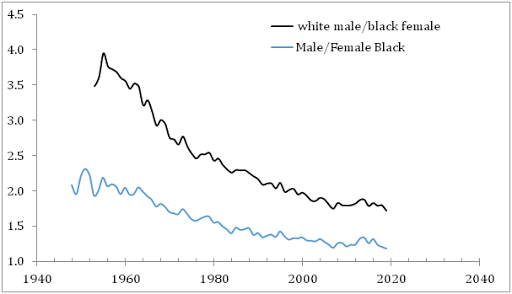## 2/5/12

### Krugman and damned lies about income inequality. No politics

Paul Krugman and a bigger company have been speculating on the increasing economic inequality in the US.  They do not trust any data from the BLS (income measurements obtained during Current Population Surveys) and deny that income data from censuses can be used to characterize Gini coefficient since these data sets do not contain higher incomes. They claim that the most interesting processes have been evolving at very high incomes.  In this post, I am going to justify the estimates of Gini reported by the BLS.  My goal is to extend the distribution of personal incomes to as high level as possible and to demonstrate that this distribution follows up the Pareto distribution, i.e. is well described by a simple power law. This observation allows replacing (interpolate) actual measurements with a simple function when calculating the Lorenz curve and thus Gini coefficient.

Following this direction, we have recently reported that the personal income distribution, PID,  in the USA does not change with time when normalized to the total population and total income. In other words, the relative distribution of personal income in the United States has not been changing since the start of income measurements in 1947. The accuracy of early measurements is not good enough, however, and we have to rely of the most recent results.
The US Census Bureau routinely reports income estimates obtained during the Annual Social and Economic Supplement of the Current Population Surveys. We begin with the higher income range as reported by the BLS and have retrieved the population distribution over mean income in the range from \$0 to \$250,000. These distributions are available only from 2000. The relevant measurements of the number of people in a given income range were carried out in \$2500 bins between \$0 and \$100,000 and \$50000 bins between \$100,000 and \$250,000.
The personal income distributions, as reported by the BLS in current dollars, are affected by the change in population (working age population), and nominal GDP growth. Also the width of income bins varies with income level. Therefore, one cannot directly compare PIDs obtained in different years. In order to suppress the influence of the width we have calculated the population density, i.e. the ratio of the number of people in a given bin and its width. Since the personal income is measured in current dollars we have to reduce all incomes by the total change of the GDP deflator since 2000 to a given year. Figure 1 shows the result of normalization for 2000, 2005, and 2010. In relative terms, the income distribution has not been changing since 2000. At higher incomes, all three curves are practically identical. This observation is validated by the estimates of Gini coefficient provided by the Census Bureau. There is a high income cap of \$250,000 (all incomes above the cap are gather in one group), which is used by Krugman and company to deny the BLS estimates.
Let’s take a look the data they used to prove the increasing inequality. The IRS measured incomes are usually referred to.  Without loss of generality, we have retried “Table 1.1 Selected Income and Tax Items, by Size and Accumulated Size of Adjusted Gross Income, Tax Year 2009”. (Any other year between 1996 and 2009 is good as well.) This Table lists individual incomes in various income bins from \$1 to \$10,000,000. There are also 8274 reports of income above \$10,000,000. We cannot use the latter incomes but definitely can plot the population density function for all incomes below \$10,000,000. Figure 2 depicts the whole PID and Figure 3 its high income portion. The higher incomes are well approximated by a power low with an exponent of -3.07. (The difference of ~1.0 from the exponent for the BLS PDF (-4.1) is completely explained by the normalization to the total personal income reported by the BLS. It means that both exponents are identical.) It is likely that the same power law is valid at incomes higher than \$10,000,000. Hence, there is no significant deviation (except measurement errors) from the Pareto distribution even at very high incomes and our extrapolation of the BLS incomes along the power law is valid for the calculations of Gini coefficients.

Conclusion: there is no growth in income inequality.  Krugman et al. definitely exaggerate. As a Russian physicist, I have no political or any other emotional prejudice to the income distribution in the USA. I just calculate it.

Figure 1. The population density function, PDF, as a function of mean income as normalized to the total personal income for a given year. At higher incomes, the curves are practically identical.

Figure 2. Population density function reported by the IRS.

Figure 3. Population density function reported by the IRS for high incomes. The Pareto distribution is obvious.  Fluctuations are likely related to measurement error.

### The mean income gap between white males and black females grows during the democratic presidencies

Two days ago, we compared the mean income evolution of the white and black population and demonstrated that the difference did not change mu...